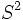# Chow's theorem

Let$S^2$ denote the 2-sphere (upto differential structure) and$g$ any Riemannian metric on$S^2$. Then, the Ricci flow on$S^2$ starting from$g$, becomes positive in finite time.
This, along with Hamilton's theorem on Ricci flows, gives the Ricci flow convergence theorem on compact surfaces which states that any Ricci flow starting from a Riemannian metric on a compact surface converges, at time$\infty$, to a constant-curvature metric.